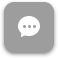Call Now 855-300-1469

# MTH 1703 Finite Mathematics

#### Course Description

In this course, a student will be exposed to topics in mathematics that are especially applicable to business, such as linear models, linear programming, mathematics of finance, counting methods, probability, and statistics

#### Course Objectives

Upon completion of this course, students should be able to

• To prepare for courses of study in finance, statistics, quantitative methods, accounting, marketing, economics, and computer information systems
• Successfully answer questions pertaining to certain topics included in the CLAST exams
• Use quantitative reasoning to enhance decision-making capability

### Week 1

##### Lecture: Set Theory

Outcomes

• Discuss the basic concepts of set theory
• Use three methods to represent sets
• Determine whether two sets are equal or equivalent
##### Lecture: Venn Diagram

Outcomes

• Discuss the meaning of a universal set
• Determine the number of subsets of a set
• Perform operations with sets
• Use Venn diagrams to illustrate equality of sets

### Week 2

##### Lecture: Algebra Review

Outcomes

• Evaluate algebraic expressions
• Simplify algebraic expressions
• Evaluate formulas
##### Lecture: Linear Equations and Inequalities

Outcomes

• Solve linear equations
• Solve for a variable in an equation or formula
• Solve a “word” problem using linear equations
• Graph the solutions of an inequality on a number line
• Solve applied problems using linear inequalities

### Week 3

##### Lecture: Graphs and Functions

Outcomes

• Graph equations
• Use the f(x) notation
• Use intercepts to graph a linear equation
• Calculate the slope of a line
• Interpret slope and y-intercept in applied situations
##### Lecture: Linear Systems

Outcomes

• Solve linear systems by graphing, substitution, and addition
• Solve “word” problems using systems of linear equations
• Graph a system of linear inequalities

### Week 4

##### Lecture: Consumer Mathematics and Financial Management - Part 1

Outcomes

• Solve applied problems involving percent
• Calculate simple interest and maturity value
• Use compound interest formulas
• Calculate present value
• Understand and compute effective annual yield
• Find the value of an annuity
##### Lecture: Consumer Mathematics and Financial Management - Part 2

Outcomes

• Determine the amount financed, the installment price, and the finance charge for a fixed loan
• Compute unearned interest and the payoff amount for a loan paid off early
• Find the interest, balance due, and minimum monthly payment for credit card loans
• Compute interest costs for a mortgage
• Recognize stocks, bonds, and mutual funds as investments

### Week 5

##### Lecture: Counting Methods and Probability Theory

Outcomes

• Use the Fundamental Counting Principle to determine the number of possible outcomes in a given situation
• Use the permutations formula
• Use the combinations formula
• Distinguish between permutation and combination problems
• Compute theoretical and empirical probability
• Compute probabilities with permutations
• Compute probabilities with combinations

### Week 6

##### Lecture: Odds, Conditional Probability, and Expected Value

Outcomes

• Determine and use odds
• Compute conditional probabilities
• Use expected value to determine the average payoff or loss in a game of chance

### Week 7

##### Lecture: Statistics

Outcomes

• Describe the population whose properties are to be analyzed
• Organize and present data
• Identify deceptions in visual displays of data
• Calculate the measures of central tendency
• Determine the standard deviation for a data set

### Week 8

##### Lecture: Normal Distribution

Outcomes

• Recognize the characteristics of normal distributions
• Convert a data item to a z-score
• Identify and use percentiles
• Use and interpret margins of error

The course description, objectives and learning outcomes are subject to change without notice based on enhancements made to the course. November 2011